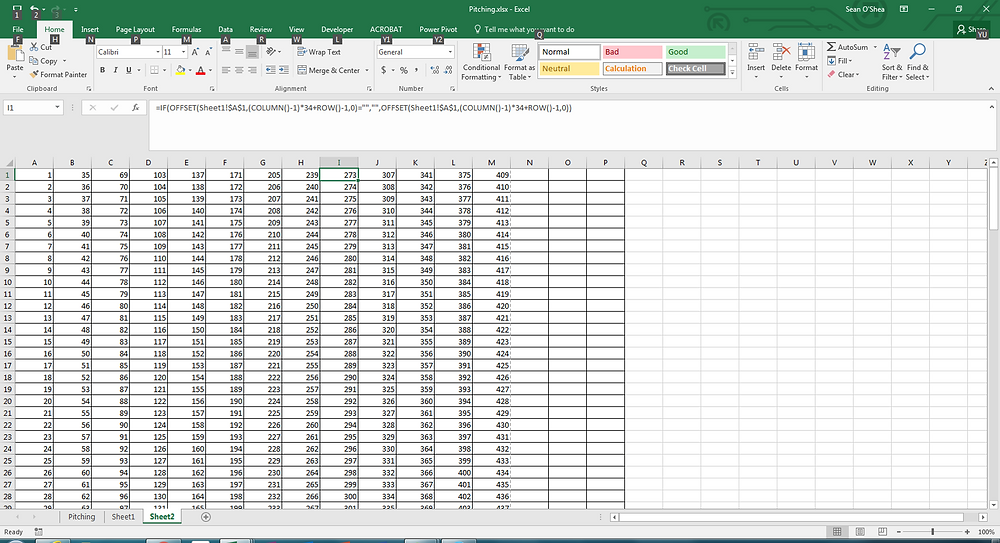Search

# Snaking Columns in Excel

When you're preparing an exhibit with a single column of data it may be very helpful to have the data snake across the pages. So rather than one column simply appearing at the left of each page, you can have the column shift over to the right when it reaches the bottom of the page, and keep shifting until it reaches the far right of the page. Using this technique the overall page count of the exhibit can be reduced greatly.

In this example (where we use sequential number for the sack of clarity), we begin with a 1000 numbers listed in column A of an Excel spreadsheet. As you can see from this example, in landscape mode, it will take up 30 pages.On a new worksheet we enter this formula in cell A1 which references the data on the first worksheet:

=IF(OFFSET(Sheet1!\$A\$1,(COLUMN()-1)*34+ROW()-1,0)="","",OFFSET(Sheet1!\$A\$1,(COLUMN()-1)*34+ROW()-1,0))The formula is set to put 34 rows on a single page, but if you want to re-set your margins to accommodate more rows, you can change the 34's in the formula to some other number. Just pull the formula down using the fill handle to row 34, and then pull the formula across 8 or 9 columns.

When you want the entries to continue snaking on the next page, modify the formula to change the references to A1 to the cell from the first worksheet is shown in the last cell listed on the first page of the second worksheet - not the cell which you want to be displayed first on the second worksheet. Then change the end of the formula from -1,0)) to -34,0)) , so the formula looks like this:

=IF(OFFSET(Sheet1!\$A\$442,(COLUMN()-1)*34+ROW()-1,0)="","",OFFSET(Sheet1!\$A\$442,(COLUMN()-1)*34+ROW()-34,0))

The result is a list that takes up only 3 pages instead of 30. Note that in order to get this formula to function for your complete list, you may need to append additional entries to the end of your list on the first worksheet.

This formula will work with text entries as well as numbers.